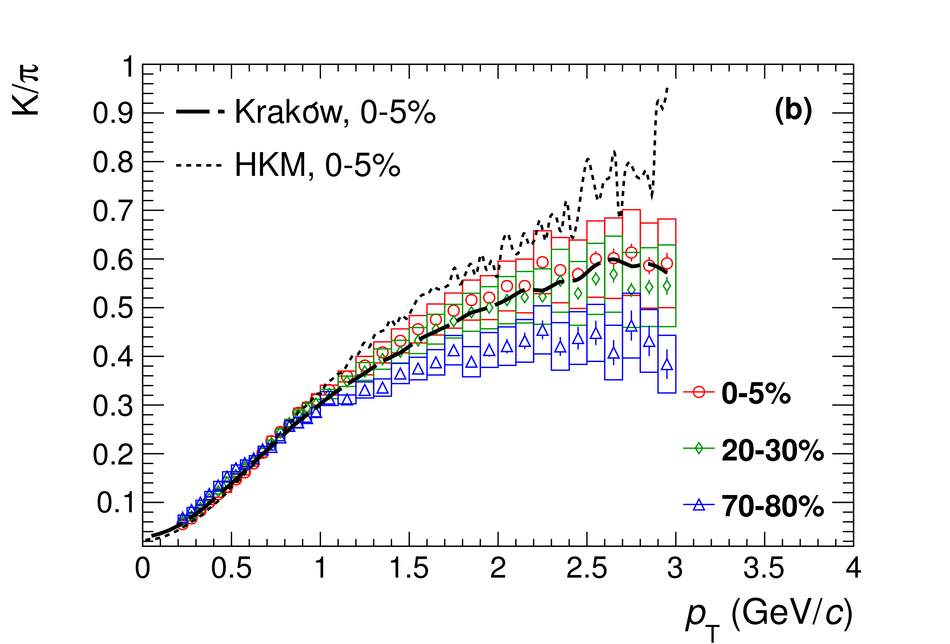# Figure 16

 (a) $\rm{p}/\pi = (\mathrm{p} +\mathrm{\bar{p}})/(\pi^++\pi^-)$ as a function of $\pt$ for different centrality bins compared to ratios from the Krak'ow and HKM hydrodynamic models and to a recombination model; (b) $\rm{K}/\pi = (\mathrm{K^+} + \mathrm{K^-}))/(\pi^++\pi^-)$ ratio as a function of $\pt$ for different centrality bins compared to ratios from the Krak'ow and HKM hydrodynamic models.